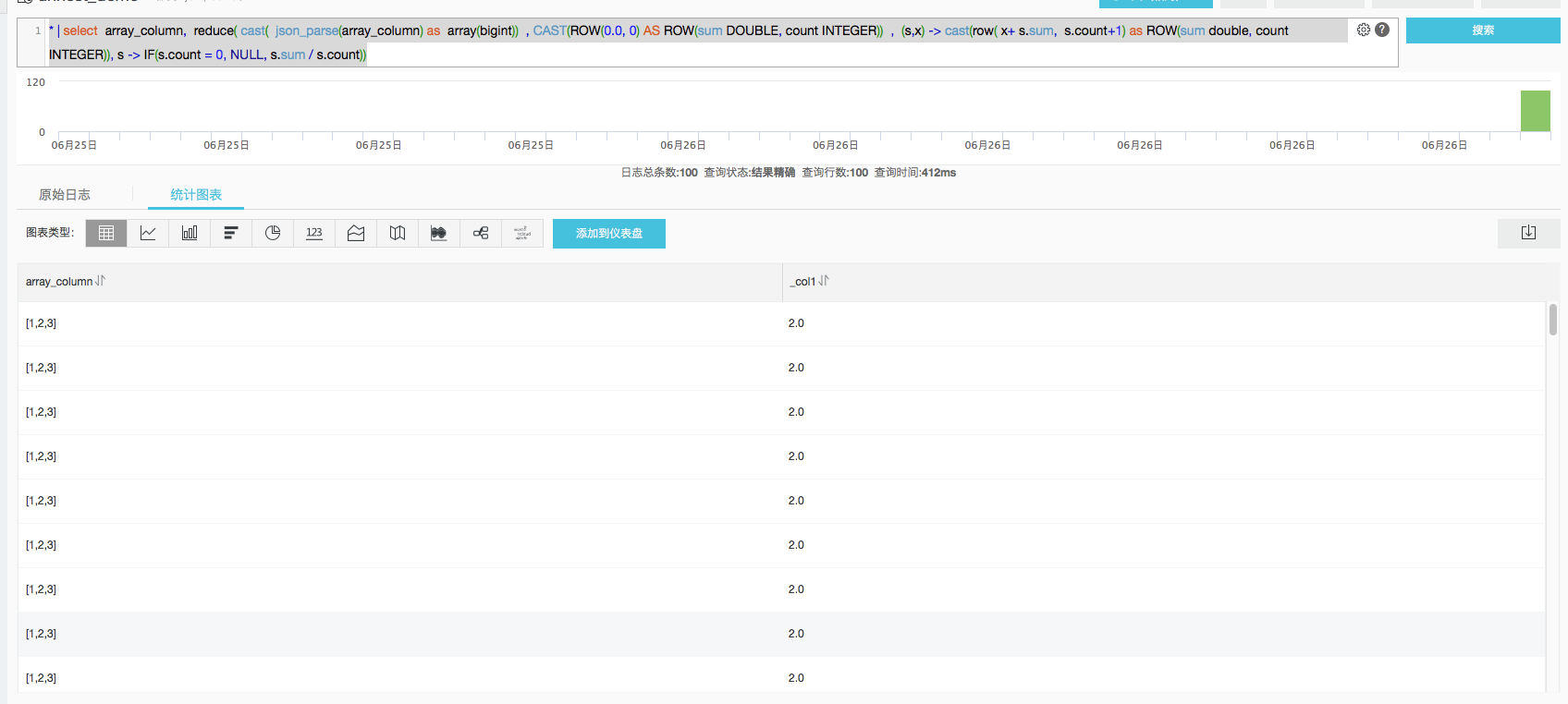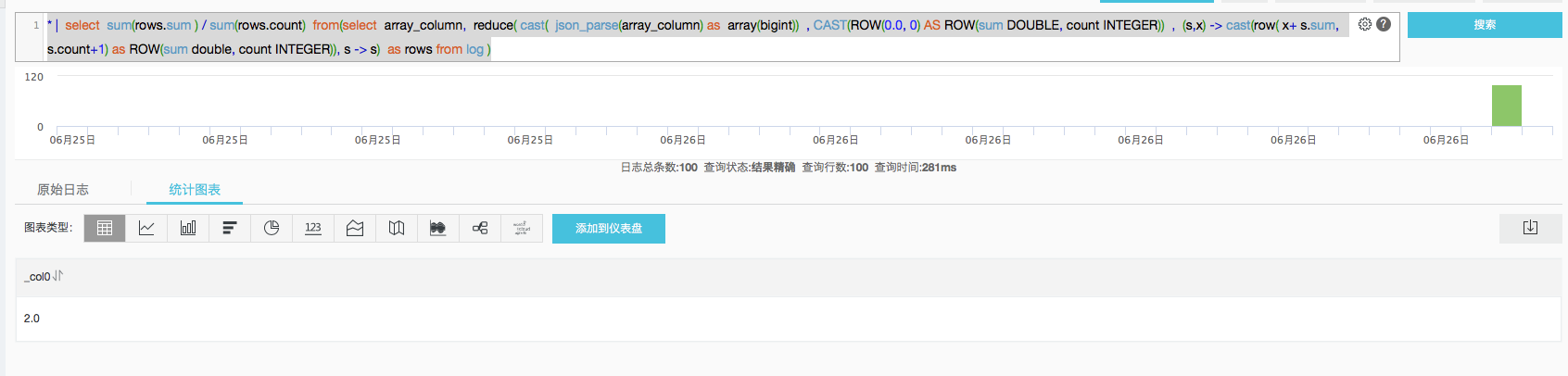#1. 云栖社区>
2. 阿里云存储服务>
3. 博客>
4. 正文

## 场景

``````__source__:  11.164.232.105
__tag__:__hostname__:  vm-req-170103232316569850-tianchi111932.tc
__topic__:  TestTopic_4
array_column:  [1,2,3]
double_column:  1.23
map_column:  {"a":1,"b":2}
text_column:  商品``````

## lambda函数对array类型的数据进行求均值

``* | select  array_column,  reduce( cast(  json_parse(array_column) as  array(bigint))  , CAST(ROW(0.0, 0) AS ROW(sum DOUBLE, count INTEGER))  ,  (s,x) -> cast(row( x+ s.sum,  s.count+1) as ROW(sum double, count INTEGER)), s -> IF(s.count = 0, NULL, s.sum / s.count))``reduce 函数的具体语义参考语法文档。参数分为四部分

1. `cast( json_parse(array_column) as array(bigint))` 表示输入的数组数据
2. `CAST(ROW(0.0, 0) AS ROW(sum DOUBLE, count INTEGER))` 定义起始状态为一个复杂的row类型，分别记录sum和count
3. 对每一个元素，计算累加值，`(s,x) -> cast(row( x+ s.sum, s.count+1) as ROW(sum double, count INTEGER))` s代表已经有的状态，x代表新输入的元素，计算结果通过cast强制定义为row类型
4. 最后对最终状态，计算avg值，`s -> IF(s.count = 0, NULL, s.sum / s.count)`。s代表最终状态。

## 对所有行的array元素求avg：

``````* |  select  sum(rows.sum ) / sum(rows.count)  from(
select  array_column,  reduce( cast(  json_parse(array_column) as  array(bigint))  , CAST(ROW(0.0, 0) AS ROW(sum DOUBLE, count INTEGER))  ,  (s,x) -> cast(row( x+ s.sum,  s.count+1) as ROW(sum double, count INTEGER)), s -> s)  as rows from log
)``````